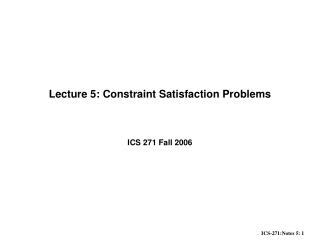# Lecture 5: Constraint Satisfaction Problems - PowerPoint PPT PresentationDownload PresentationLecture 5: Constraint Satisfaction Problems

Lecture 5: Constraint Satisfaction ProblemsDownload Presentation## Lecture 5: Constraint Satisfaction Problems

- - - - - - - - - - - - - - - - - - - - - - - - - - - E N D - - - - - - - - - - - - - - - - - - - - - - - - - - -
##### Presentation Transcript

1. Lecture 5: Constraint Satisfaction Problems ICS 271 Fall 2006

2. Outline • The constraint network model • Variables, domains, constraints, constraint graph, solutions • Examples: • graph-coloring, 8-queen, cryptarithmetic, crossword puzzles, vision problems,scheduling, design • The search space and naive backtracking, • The constraint graph • Consistency enforcing algorithms • arc-consistency, AC-1,AC-3 • Backtracking strategies • Forward-checking, dynamic variable orderings • Special case: solving tree problems • Local search for CSPs

3. E A B red green red yellow green red green yellow yellow green yellow red A D B F G C Constraint Satisfaction Example: map coloring Variables - countries (A,B,C,etc.) Values - colors (e.g., red, green, yellow) Constraints:

4. Sudoku • Variables: 81 slots • Domains = {1,2,3,4,5,6,7,8,9} • Constraints: • 27 not-equal Constraint propagation 2 34 6 2 Each row, column and major block must be alldifferent “Well posed” if it has unique solution: 27 constraints

5. Examples • Cryptarithmetic • SEND + • MORE = MONEY • n - Queen • Crossword puzzles • Graph coloring problems • Vision problems • Scheduling • Design

6. 4 2 Example 1: The 4-queen problem • Standard CSP formulation of the problem: • Variables: each row is a variable. Place 4 Queens on a chess board of 4x4 such that no two queens reside in the same row, column or diagonal. 1 2 3 4 Q Q Q Q Q Q Q Q Q Q Q Q • Domains: ( ) • Constraints: There are = 6 constraints involved: • Constraint Graph :

7. The search space • Definition: given an ordering of the variables • a state: • is an assignment to a subset of variables that is consistent. • Operators: • add an assignment to the next variable that does not violate any constraint. • Goal state: • a consistent assignment to all the variables.

8. The search space depends on the variable orderings

9. Search space and the effect of ordering

10. Backtracking • Complexity of extending a partial solution: • Complexity of consistent O(e log t), t bounds tuples, e constraints • Complexity of selectvalue O(e k log t)

11. A coloring problem

12. Backtracking search

13. Line drawing Interpretations

14. Class scheduling/Timetabling

15. The Minimal network:Example: the 4-queen problem

16. Approximation algorithms • Arc-consistency (Waltz, 1972) • Path-consistency (Montanari 1974, Mackworth 1977) • I-consistency (Freuder 1982) • Transform the network into smaller and smaller networks.

17. Arc-consistency X Y  1, 2, 3 1, 2, 3 1  X, Y, Z, T  3 X  Y Y = Z T  Z X  T  = 1, 2, 3 1, 2, 3  T Z

18. 1 3 2 3 Arc-consistency X Y  1  X, Y, Z, T  3 X  Y Y = Z T  Z X  T  =  T Z • Incorporated into backtracking search • Constraint programming languages powerful approach for modeling and solving combinatorial optimization problems.

19. Arc-consistency algorithm domain of x domain of y Arc is arc-consistent if for any value of there exist a matching value of Algorithm Revise makes an arc consistent Begin 1. For each a in Di if there is no value b in Dj that matches a then delete a from the Dj. End. Revise is , k is the number of value in each domain.

20. Algorithm AC-3 • Complexity • Begin • 1. Q <--- put all arcs in the queue in both directions • 2. While Q is not empty do, • 3. Select and delete an arc from the queue Q • 4. Revise • 5. If Revise cause a change then add to the queue all arcs that touch Xi (namely (Xi,Xm) and (Xl,Xi)). • 6. end-while • end • Processing an arc requires O(k^2) steps • The number of times each arc can be processed is 2·k • Total complexity is

21. Example applying AC-3

22. Sudoku Each row, column and major block must be alldifferent “Well posed” if it has unique solution

23. Sudoku Each row, column and major block must be alldifferent “Well posed” if it has unique solution

24. Sudoku • Variables: 81 slots • Domains = {1,2,3,4,5,6,7,8,9} • Constraints: • 27 not-equal Constraint propagation 2 34 6 2 Each row, column and major block must be alldifferent “Well posed” if it has unique solution: 27 constraints

25. Sudoku Each row, column and major block must be alldifferent “Well posed” if it has unique solution

26. Schemes for improving backtracking • Before search: (reducing the search space) • Arc-consistency, path-consistency • Variable ordering (fixed) • During search: • Look-ahead schemes: • value ordering, • variable ordering (if not fixed) • Look-back schemes: • Backjump • Constraint recording • Dependency-directed backtacking

27. Look-ahead: Variable and value orderings • Intuition: • Choose value least likely to yield a dead-end • Choose a variable that will detect failures early • Approach: apply propagation at each node in the search tree • Forward-checking • (check each unassigned variable separately • Maintaining arc-consistency (MAC) • (apply full arc-consistency)